### Geometry Fundamentals (2019)

We've seen some ways we can explore the side lengths and angles of shapes. Another way we'll measure size of a shape is by the area it covers.

These three techniques are critical, and they're flexible strategies, not formulas.

1. We can draw in lines or even a whole grid to cut up shapes and patterns of shapes.

2. After cutting up a shape, we can rearrange the pieces so that calculating the area becomes simply a matter of counting squares.

3. We can sometimes make and then rearrange extra copies of a shape so that all of the pieces combined can make a single square or rectangle.

The big theme here is that rectangles and squares are key because we can find their areas very quickly!

# Working with Areas

In this $9$ by $6$ rectangle, is more area shaded or not shaded?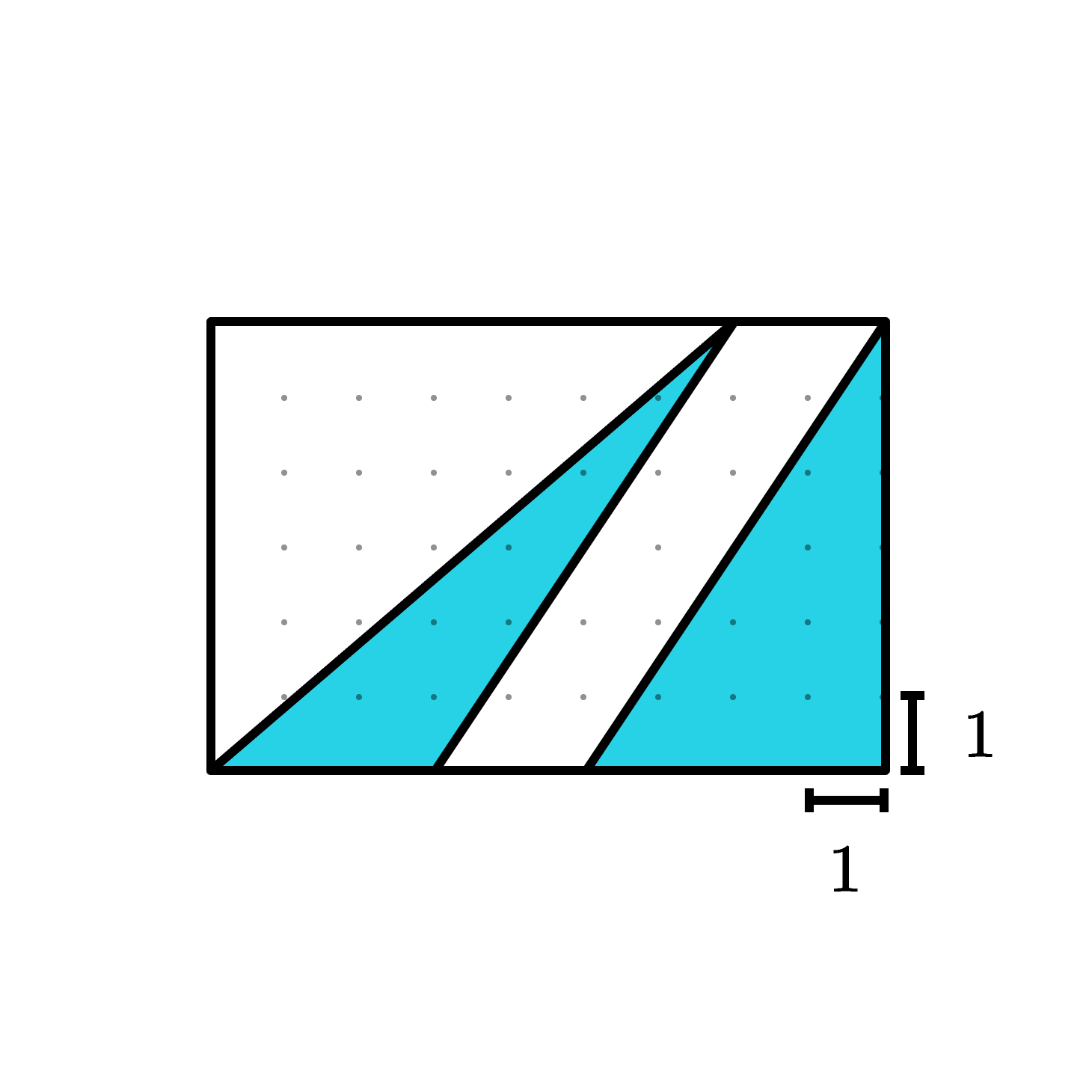# Working with Areas

What fraction of the rectangle's area is taken up by the regular hexagon?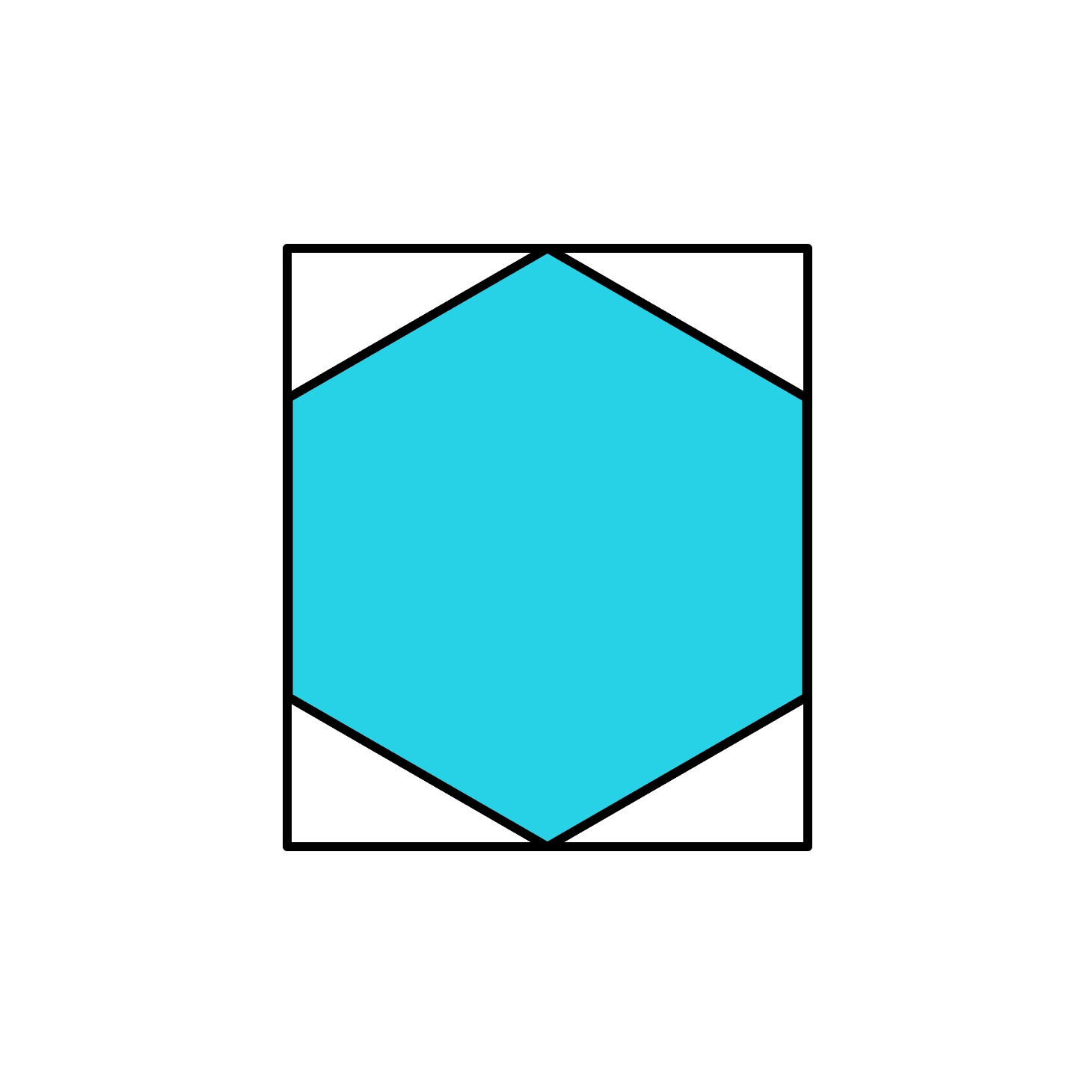# Working with Areas

What is the area of the shaded part of this $8$ by $5$ rectangle?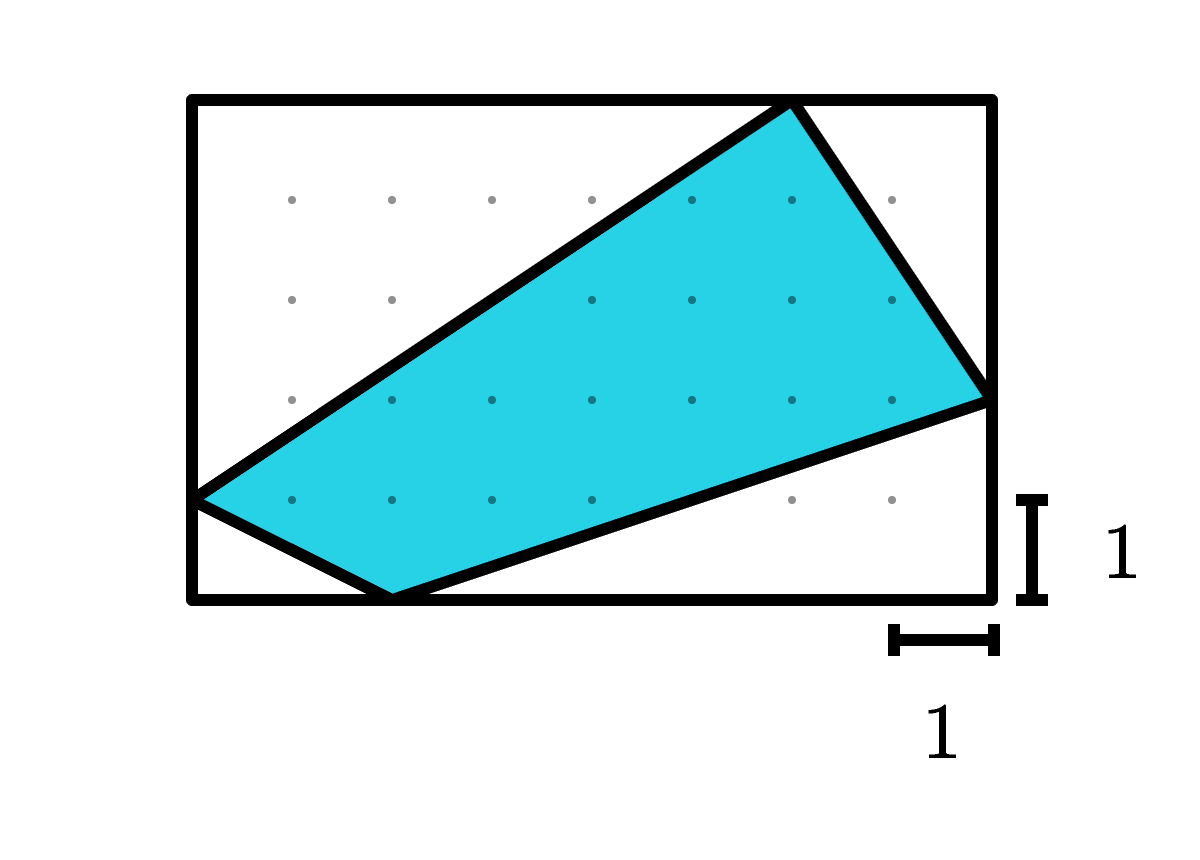# Working with Areas

Even when shapes have curved sides, we can still reason with and compare their areas.

The centers of the circles and a corner of the square are all at the same point. The square's side length is $10 \text{ m}.$

What is the area of the shaded part of the diagram?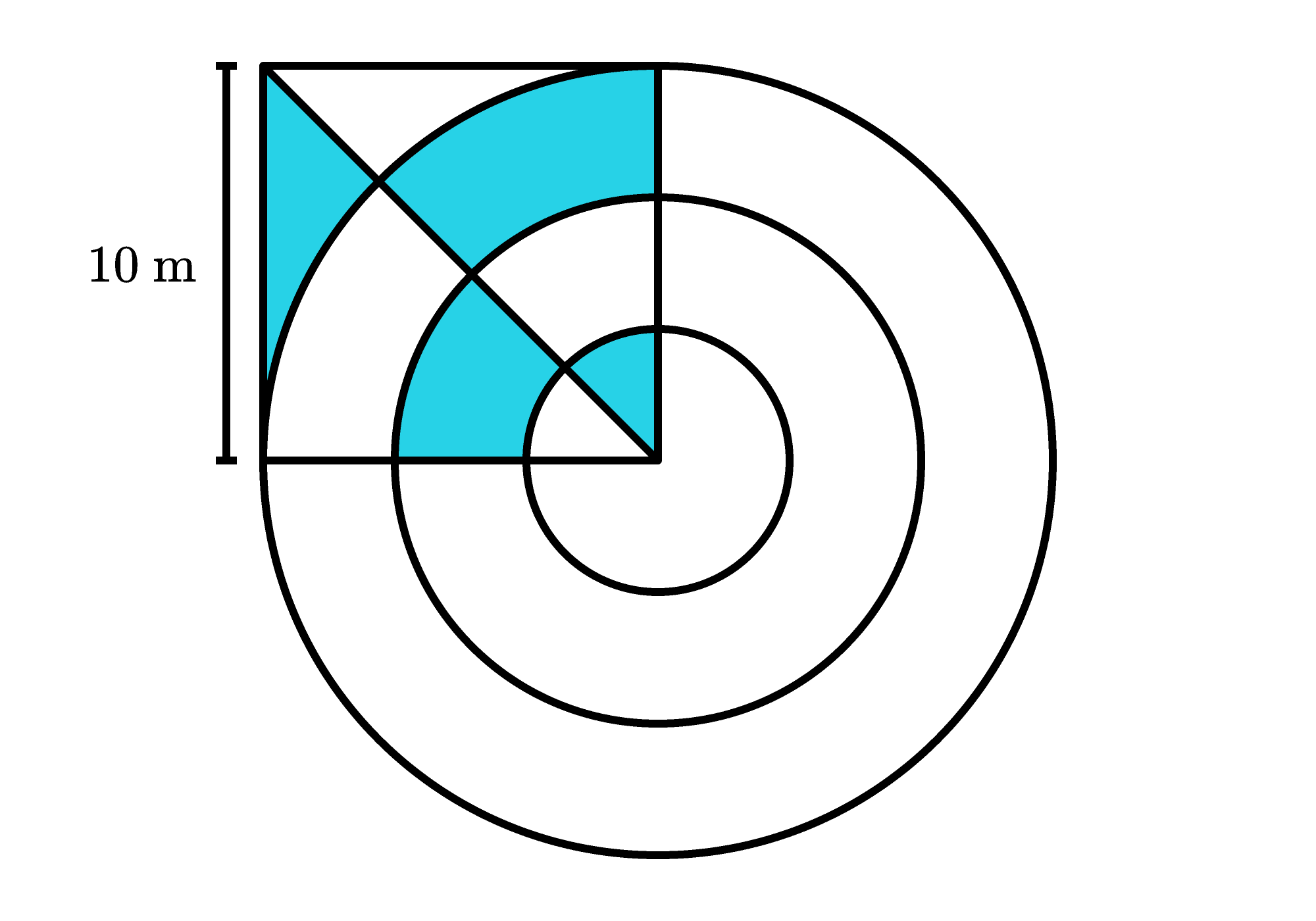# Working with Areas

Which figure has the largest shaded area?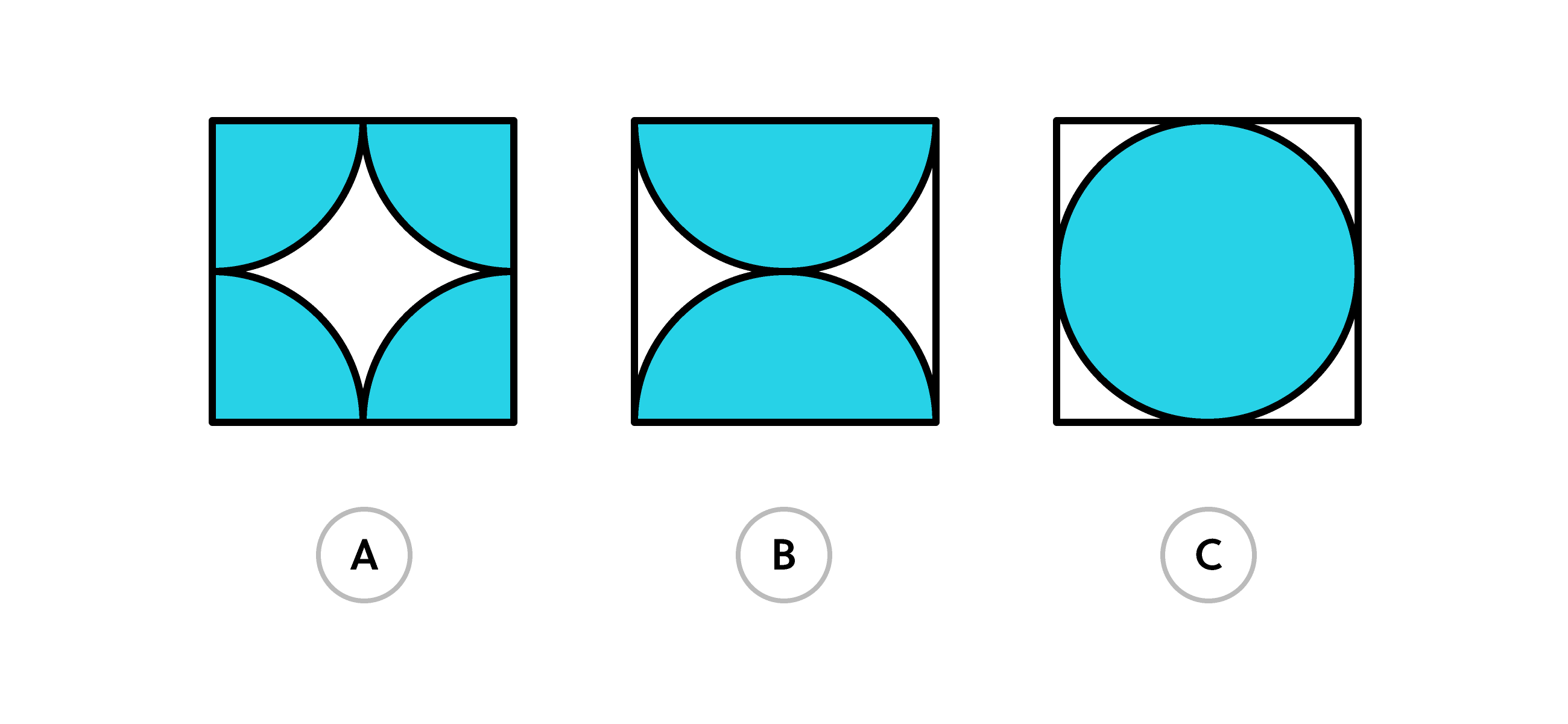When solving this problem, you can assume that:

• The three squares above are all the same size.
• Each curve in the three figures is a circle segment from a circle with diameter equal to the side length of the square.

# Working with Areas

We've just seen a preview of some ways we can reason with squares, rectangles, and triangles to help think about the areas of shapes. By cutting up and rearranging pieces of figures, we can change them into more familiar shapes without changing their areas. These are ideas that we'll come back to later in this course, even applying them to 3D shapes!

×